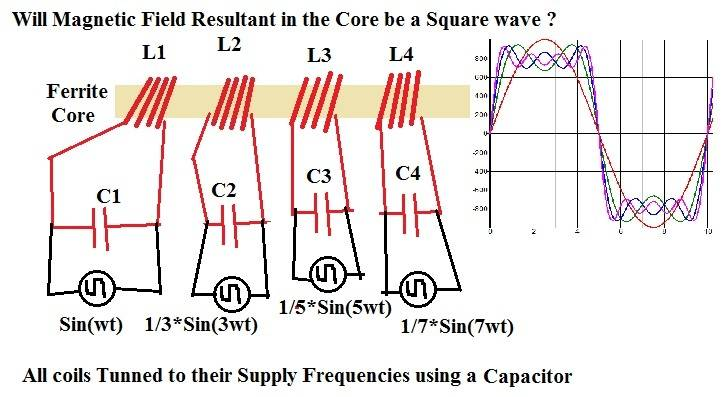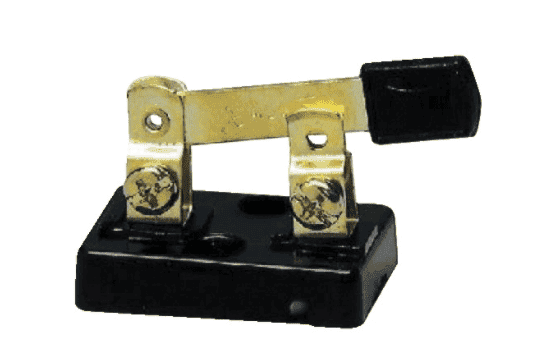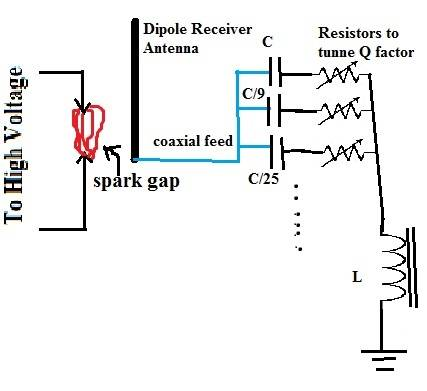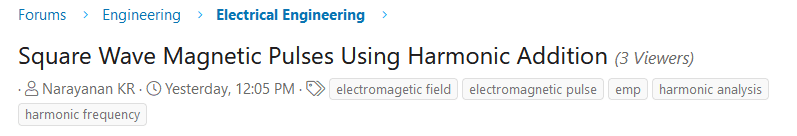# Square Wave Magnetic Pulses Using Harmonic Addition

Summary:
Fourier Series Expansion says that we can get square wave pulses by adding a sinusoidal wave with its odd order harmonics attenuated by factor proportional to frequency of the Harmonic. Applying this Technique to Inductors (or Transformers) as shown in the Circuit Diagram below, Can we get nearly perfect square wave magnetic pulses which will then have a lot of practical uses ? If this method is not the proper way, then how else would we achieve square waves ?berkeman
Mentor
First, you would have to line up the phases of your different sine wave generators...

Second, the first "tuned" coil drive circuit is only tuned when there are no other "tuned" circuits on the common core. Adding them presents a different impedance to the driven coil circuit, which messes up the tuning. If you remove the tuning capacitors and just have the real output impedances of the sine wave generators, that might work better.

DaveE
Gold Member
Theoretically yes (if you listen to what @berkeman has said). But why? Why do it this way? It is really easy to generate square waves with switching circuits. This is how most switching power supplies work.

OK, this is really "out of left field" but it reminds me of pre-compensation in digital signal transmission. Your magnetic structure will have its own frequency response, So, just because Mr. Fourier says what the amplitudes of the harmonics should be at the output, that doesn't mean that that's the same as what you will need to create at the input. For example, to get a nice square wave out of a low pass filter, you will want to overdrive the high frequency harmonics.

anorlunda
Staff Emeritus
If this method is not the proper way, then how else would we achieve square waves ?

Open-close-open-close-open-close ...Or, use transistors to do the switching for you at high speed.

•sophiecentaur
Open-close-open-close-open-close ...
View attachment 289405

Or, use transistors to do the switching for you at high speed.
By using a simple switch or transistor only voltage across the inductor can be made square, the current and hence the magnetic flux will remain triangular, my question is how to get square wave or a nearly square wave magnetic flux out ot an inductor.

DaveE
Gold Member
Open-close-open-close-open-close ...
View attachment 289405

Or, use transistors to do the switching for you at high speed.
I recall a set up I made in the 1980's to make a high current step function. It was a soft copper block covered with a piece of tissue paper. Then I had another metal spike with a sharp point, both connected to wire braid. When I hit it with a hammer I got a surprisingly fast rise time.

•anorlunda
hutchphd
Homework Helper
I guess a square wave could get pretty noisy:

•Narayanan KR
Baluncore
You cannot produce a square wave magnetic flux because that requires a finite energy transfer in an infinitesimal time.
That requires infinite power conversion into magnetic flux. E= ½·L·I².
A similar problem is to produce a square voltage waveform across a capacitor. E= ½·C·V².
Mechanically it requires infinite acceleration to produce a square wave in velocity. E= ½·m·v².

•DaveE
Gold Member
You cannot produce a square wave magnetic flux because that requires a finite energy transfer in an infinitesimal time.
That requires infinite power conversion into magnetic flux. E= ½·L·I².
A similar problem is to produce a square voltage waveform across a capacitor. E= ½·C·V².
Mechanically it requires infinite acceleration to produce a square wave in velocity. E= ½·m·v².
OK, I think everyone but you assumed it was bandwidth limited. Also I suspect the OP knows that he can't provide the infinite number of harmonics the Fourier sequence requires. I think that may be why he said "nearly perfect square wave".

But, you are correct. We all agree 100%.

Baluncore
OK, I think everyone but you assumed it was bandwidth limited.
No. I knew it must be bandwidth limited.
To you and me it may be obvious, but I believed the limit to the approximation of the impossible needed to be clearly stated.

•Narayanan KR
No. I knew it must be bandwidth limited.
To you and me it may be obvious, but I believed the limit to the approximation of the impossible needed to be clearly stated.
Now I understand why my initial idea is not proper, but again If I want an approximate square wave, can i use the below circuit.
Here the spark gap produces noises at all frequencies, also a dipole antenna will not only receive a quater wave but also its odd ordered harmonics (3rd, 5th, 7th e.t.c because dipole antenna is a poly-resonant circuit), then if i use capacitors and resistors to tune the current flow into the inductor, then will all those currents add up to give an approximate square wave as expected initially ?•Baluncore
berkeman
Mentor
my question is how to get square wave or a nearly square wave magnetic flux out ot an inductor.
What's the derivative of a square wave? Or more to the point, what is the derivitave of a trapezoidal wave with steep rise and fall times?

Baluncore
... then will all those currents add up to give an approximate square wave as expected initially ?
Unfortunately not. Phase will again be a problem, and the broad-band noise energy will have little energy at the narrow-band harmonics required.

To produce an approximate square wave flux you need an approximate square wave current, ±i, in the flux inductor. That ±i can be generated by combining two techniques. First the inductor has series resistance, Rs, so you drive it with a square wave of voltage V = i * Rs. That will settle exponentially towards ±i following each transition. Next, that exponential hole must be filled by a high energy voltage spike that will change the current direction as quickly as possible, then drop back at the same exponential rate to give the wave a flat top. Current could be built up in a separate inductor, then switched at the instant of transition into the flux inductor. Maybe that could be done through a secondary winding on the flux inductor.

••DaveE and Narayanan KR
Unfortunately not. Phase will again be a problem, and the broad-band noise energy will have little energy at the narrow-band harmonics required.

To produce an approximate square wave flux you need an approximate square wave current, ±i, in the flux inductor. That ±i can be generated by combining two techniques. First the inductor has series resistance, Rs, so you drive it with a square wave of voltage V = i * Rs. That will settle exponentially towards ±i following each transition. Next, that exponential hole must be filled by a high energy voltage spike that will change the current direction as quickly as possible, then drop back at the same exponential rate to give the wave a flat top. Current could be built up in a separate inductor, then switched at the instant of transition into the flux inductor. Maybe that could be done through a secondary winding on the flux inductor.
hmm..now my ideas sound less practical, One last doubt though, isn't this suqare wave magnetic pulse already achieved in a Two Cavity Klystron Amplifier Tube, where the velocity modulated electrons (fast, reference and slow) all arrive at the output in one instant creating a bunching effect, whose associated magnetic field could resemble a square wave impulse ?

Baluncore
There are ways to sharpen steep edges using Non-Linear Transmission Lines and pulse shaping networks.

Maybe if you explained why you need a square flux wave it would be possible to find an easier solution. What pulse repetition frequency and risetime do you require ?

•Narayanan KR
berkeman
Mentor
Maybe if you explained why you need a square flux wave it would be possible to find an easier solution. What pulse repetition frequency and risetime do you require ?
The tags he added to this thread may offer a clue. He has been known to post asking how to generate an EMP. I haven't complained in this thread so far, because he was presumably talking about low-power signal generators as the sources...hutchphd
Homework Helper
I don't see a big problem here.....

There are ways to sharpen steep edges using Non-Linear Transmission Lines and pulse shaping networks.

Maybe if you explained why you need a square flux wave it would be possible to find an easier solution. What pulse repetition frequency and risetime do you require ?
lets say that i have 10 Mhz 5 V peak to peak sine wave, here the "raise time" will be one quarter of time period (100 nS / 4 = 25 nS), can I use your suggested nonlinear transmission line to reduce the raise time by half ? v.i.z 12.5 nS, is the frequency I chose too high ?

Baluncore
I don't see a big problem here.....
I don't see a problem either. EMP generators do not need flat tops, they only need a massive discharge current. It is all very well reading about them, but building one is simply not a sensible pastime. If the OP had the budget, time and the skill needed to build an effective one, his door would be kicked in about an hour later.

The traditional Marx Pulse Generator produces a local high voltage lightning strike without too much radio interference. They are best operated in screened rooms and used for testing insulation and lightning strike survivability.
https://en.wikipedia.org/wiki/Marx_generator

The current equivalent of the Marx Generator produces a circular current that will destroy nearby semiconductor electronics and wipe out the radio spectrum. It will rapidly draw attention from the authorities as the pulses show up as very unusual repeated lightning strikes on the map at the national lightning monitoring networks.
“A Novel Type of High Power Pulse Transmitter”. By K. Landecker, and K. S. Imprie. 1960.
https://www.publish.csiro.au/ph/pdf/ph600638

Homopolar generators have been built to generate currents for magnetic pulses. The energy and currents are massive, but the RF interference is contained.
https://en.wikipedia.org/wiki/Homopolar_generator#Homopolar_generator_development

•DaveE and hutchphd
Baluncore
lets say that i have 10 Mhz 5 V peak to peak sine wave, here the "raise time" will be one quarter of time period (100 nS / 4 = 25 nS), can I use your suggested nonlinear transmission line to reduce the raise time by half ? v.i.z 12.5 nS, is the frequency I chose too high ?
That is trivial. A 10 GHz wave can be steepened to a 1 ps rise sawtooth using homebrewed NLTLs.
The faster 100 GHz oscilloscopes use NLTLs.
https://www.anritsu.com/en-us/test-measurement/technologies/shockline-nltl
https://www.embedded.com/new-sampling-technique-underpins-100-ghz-bandwidth-scopes/

•Narayanan KR
Baluncore
Different people need different references.
Use Google to search the subject.
You can simulate NLTLs using freeware such as LTspice.

•Narayanan KR
sophiecentaur